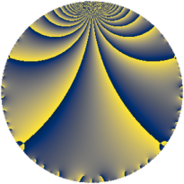# Properties

 Label 1575.2.a.cLevel $1575$ Weight $2$ Character orbit 1575.a Self dual yes Analytic conductor $12.576$ Analytic rank $1$ Dimension $1$ CM no Inner twists $1$

# Related objects

## Newspace parameters

 Level: $$N$$ $$=$$ $$1575 = 3^{2} \cdot 5^{2} \cdot 7$$ Weight: $$k$$ $$=$$ $$2$$ Character orbit: $$[\chi]$$ $$=$$ 1575.a (trivial)

## Newform invariants

 Self dual: yes Analytic conductor: $$12.5764383184$$ Analytic rank: $$1$$ Dimension: $$1$$ Coefficient field: $$\mathbb{Q}$$ Coefficient ring: $$\mathbb{Z}$$ Coefficient ring index: $$1$$ Twist minimal: no (minimal twist has level 21) Fricke sign: $$1$$ Sato-Tate group: $\mathrm{SU}(2)$

## $q$-expansion

 $$f(q)$$ $$=$$ $$q - q^{2} - q^{4} + q^{7} + 3q^{8} + O(q^{10})$$ $$q - q^{2} - q^{4} + q^{7} + 3q^{8} - 4q^{11} + 2q^{13} - q^{14} - q^{16} - 6q^{17} + 4q^{19} + 4q^{22} - 2q^{26} - q^{28} + 2q^{29} - 5q^{32} + 6q^{34} - 6q^{37} - 4q^{38} - 2q^{41} + 4q^{43} + 4q^{44} + q^{49} - 2q^{52} + 6q^{53} + 3q^{56} - 2q^{58} - 12q^{59} - 2q^{61} + 7q^{64} - 4q^{67} + 6q^{68} + 6q^{73} + 6q^{74} - 4q^{76} - 4q^{77} - 16q^{79} + 2q^{82} - 12q^{83} - 4q^{86} - 12q^{88} + 14q^{89} + 2q^{91} - 18q^{97} - q^{98} + O(q^{100})$$

## Embeddings

For each embedding $$\iota_m$$ of the coefficient field, the values $$\iota_m(a_n)$$ are shown below.

For more information on an embedded modular form you can click on its label.

Label $$\iota_m(\nu)$$ $$a_{2}$$ $$a_{3}$$ $$a_{4}$$ $$a_{5}$$ $$a_{6}$$ $$a_{7}$$ $$a_{8}$$ $$a_{9}$$ $$a_{10}$$
1.1
 0
−1.00000 0 −1.00000 0 0 1.00000 3.00000 0 0
 $$n$$: e.g. 2-40 or 990-1000 Significant digits: Format: Complex embeddings Normalized embeddings Satake parameters Satake angles

## Atkin-Lehner signs

$$p$$ Sign
$$3$$ $$-1$$
$$5$$ $$1$$
$$7$$ $$-1$$

## Inner twists

This newform does not admit any (nontrivial) inner twists.

## Twists

By twisting character orbit
Char Parity Ord Mult Type Twist Min Dim
1.a even 1 1 trivial 1575.2.a.c 1
3.b odd 2 1 525.2.a.d 1
5.b even 2 1 63.2.a.a 1
5.c odd 4 2 1575.2.d.a 2
12.b even 2 1 8400.2.a.bn 1
15.d odd 2 1 21.2.a.a 1
15.e even 4 2 525.2.d.a 2
20.d odd 2 1 1008.2.a.l 1
21.c even 2 1 3675.2.a.n 1
35.c odd 2 1 441.2.a.f 1
35.i odd 6 2 441.2.e.b 2
35.j even 6 2 441.2.e.a 2
40.e odd 2 1 4032.2.a.k 1
40.f even 2 1 4032.2.a.h 1
45.h odd 6 2 567.2.f.g 2
45.j even 6 2 567.2.f.b 2
55.d odd 2 1 7623.2.a.g 1
60.h even 2 1 336.2.a.a 1
105.g even 2 1 147.2.a.a 1
105.o odd 6 2 147.2.e.b 2
105.p even 6 2 147.2.e.c 2
120.i odd 2 1 1344.2.a.g 1
120.m even 2 1 1344.2.a.s 1
140.c even 2 1 7056.2.a.p 1
165.d even 2 1 2541.2.a.j 1
195.e odd 2 1 3549.2.a.c 1
240.t even 4 2 5376.2.c.l 2
240.bm odd 4 2 5376.2.c.r 2
255.h odd 2 1 6069.2.a.b 1
285.b even 2 1 7581.2.a.d 1
420.o odd 2 1 2352.2.a.v 1
420.ba even 6 2 2352.2.q.x 2
420.be odd 6 2 2352.2.q.e 2
840.b odd 2 1 9408.2.a.m 1
840.u even 2 1 9408.2.a.bv 1

By twisted newform orbit
Twist Min Dim Char Parity Ord Mult Type
21.2.a.a 1 15.d odd 2 1
63.2.a.a 1 5.b even 2 1
147.2.a.a 1 105.g even 2 1
147.2.e.b 2 105.o odd 6 2
147.2.e.c 2 105.p even 6 2
336.2.a.a 1 60.h even 2 1
441.2.a.f 1 35.c odd 2 1
441.2.e.a 2 35.j even 6 2
441.2.e.b 2 35.i odd 6 2
525.2.a.d 1 3.b odd 2 1
525.2.d.a 2 15.e even 4 2
567.2.f.b 2 45.j even 6 2
567.2.f.g 2 45.h odd 6 2
1008.2.a.l 1 20.d odd 2 1
1344.2.a.g 1 120.i odd 2 1
1344.2.a.s 1 120.m even 2 1
1575.2.a.c 1 1.a even 1 1 trivial
1575.2.d.a 2 5.c odd 4 2
2352.2.a.v 1 420.o odd 2 1
2352.2.q.e 2 420.be odd 6 2
2352.2.q.x 2 420.ba even 6 2
2541.2.a.j 1 165.d even 2 1
3549.2.a.c 1 195.e odd 2 1
3675.2.a.n 1 21.c even 2 1
4032.2.a.h 1 40.f even 2 1
4032.2.a.k 1 40.e odd 2 1
5376.2.c.l 2 240.t even 4 2
5376.2.c.r 2 240.bm odd 4 2
6069.2.a.b 1 255.h odd 2 1
7056.2.a.p 1 140.c even 2 1
7581.2.a.d 1 285.b even 2 1
7623.2.a.g 1 55.d odd 2 1
8400.2.a.bn 1 12.b even 2 1
9408.2.a.m 1 840.b odd 2 1
9408.2.a.bv 1 840.u even 2 1

## Hecke kernels

This newform subspace can be constructed as the intersection of the kernels of the following linear operators acting on $$S_{2}^{\mathrm{new}}(\Gamma_0(1575))$$:

 $$T_{2} + 1$$ $$T_{11} + 4$$ $$T_{13} - 2$$

## Hecke characteristic polynomials

$p$ $F_p(T)$
$2$ $$1 + T$$
$3$ $$T$$
$5$ $$T$$
$7$ $$-1 + T$$
$11$ $$4 + T$$
$13$ $$-2 + T$$
$17$ $$6 + T$$
$19$ $$-4 + T$$
$23$ $$T$$
$29$ $$-2 + T$$
$31$ $$T$$
$37$ $$6 + T$$
$41$ $$2 + T$$
$43$ $$-4 + T$$
$47$ $$T$$
$53$ $$-6 + T$$
$59$ $$12 + T$$
$61$ $$2 + T$$
$67$ $$4 + T$$
$71$ $$T$$
$73$ $$-6 + T$$
$79$ $$16 + T$$
$83$ $$12 + T$$
$89$ $$-14 + T$$
$97$ $$18 + T$$